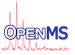OpenMS
LinearRegressionWithoutIntercept Class Reference

This class offers functions to perform least-squares fits to a straight line model, $$Y(c,x) = c_0 + c_1 x$$. More...

#include <OpenMS/MATH/STATISTICS/LinearRegressionWithoutIntercept.h>

Collaboration diagram for LinearRegressionWithoutIntercept:
[legend]

## Public Member Functions

LinearRegressionWithoutIntercept ()
Constructor. More...

void addData (double x, double y)
adds an observation (x,y) to the regression data set. More...

void addData (std::vector< double > &x, std::vector< double > &y)
adds observations (x,y) to the regression data set. More...

double getSlope () const
returns the slope of the estimated regression line. More...

## Private Attributes

double sum_xx_
total variation in x More...

double sum_xy_
sum of products More...

int n_
number of observations More...

## Detailed Description

This class offers functions to perform least-squares fits to a straight line model, $$Y(c,x) = c_0 + c_1 x$$.

## ◆ LinearRegressionWithoutIntercept()

 LinearRegressionWithoutIntercept ( )

Constructor.

## Member Function Documentation

 void addData ( double x, double y )

adds an observation (x,y) to the regression data set.

Parameters
 x independent variable value y dependent variable value

 void addData ( std::vector< double > & x, std::vector< double > & y )

adds observations (x,y) to the regression data set.

Parameters
 x vector of independent variable values y vector of dependent variable values

## ◆ getSlope()

 double getSlope ( ) const

returns the slope of the estimated regression line.

## ◆ n_

 int n_
private

number of observations

## ◆ sum_xx_

 double sum_xx_
private

total variation in x

## ◆ sum_xy_

 double sum_xy_
private

sum of products